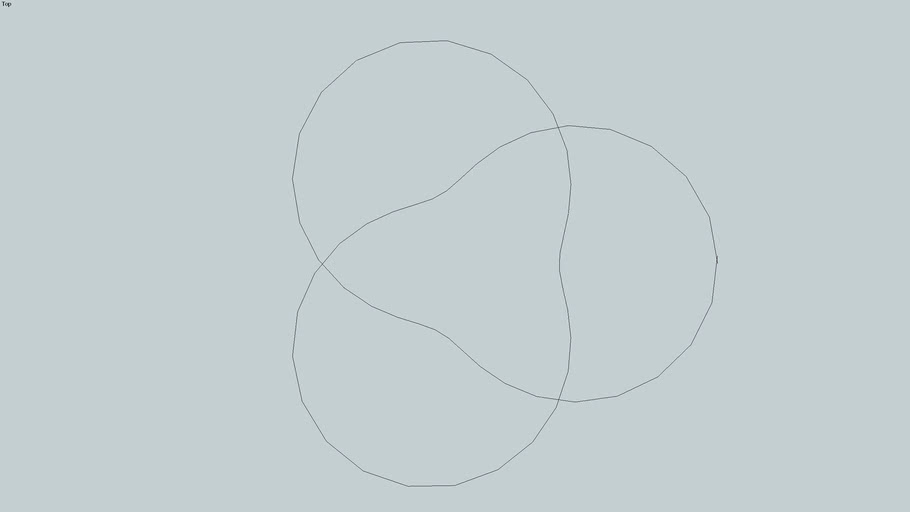# Trefoil Knot

Gregory S. Dotson
Messy but here is the ruby script to generate this shape (you can see the delimited code in the associated video): UI.menu("PlugIns").add_item("Trefoil") { # Trefoil hardcode model = Sketchup.active_model entities = model.active_entities t = 0 i = 0.2 t2 = t+i x = 0 y = 0 z = 0 x2 = 0 y2 = 0 z2 = 0 while t2 < 12.7 x = ((2+cos((3*t)/2))*cos(t)) y = ((2+cos((3*t)/2))*sin(t)) z = sin((3*t)/2) x2 = ((2+cos((3*t2)/2))*cos(t2)) y2 = ((2+cos((3*t2)/2))*sin(t2)) z2 = sin((3*t2)/2) point1 = Geom::Point3d.new (x,y,z) point2 = Geom::Point3d.new (x2,y2,z2) line = entities.add_line point1,point2 t = t + i t2 = t2 + i end } #Knot #Trefoil
Default Title